### 1. One pixel attack for fooling deep neural networks

• Motivation:
- Generating adversarial images can be formalized as an optimization problem with constraints. We assume an input image can be represented by a vector in which each scalar element represents one pixel. Let $f$ be the target image clas- sifier which receives n-dimensional inputs
- Let $f$ be the target image classifier which receives n-dimensional inputs, $\mathbf{x}=(x_1,...,x_n)$ be the original natural image correctly classified as class $t$.
- The probability of $\mathbf{x}$ belonging to the class t is therefore $f_t(\mathbf{x})$.
- The vector $e(\mathbf{x})=(e_1,...,e_n)$ is an additive adver- sarial perturbation according to $\mathbf{x}$, the target class $adv$ and the limitation of maximum modification $L$.
- Note that $L$ is always measured by the length of vector $e(\mathbf{x})$.
- The goal of adversaries in the case of targeted attacks is to find the optimized solution $e(\mathbf{x})^*$ for the following question: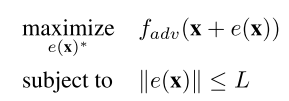• (a) which dimensions that need to be perturbed
• (b) the correspond- ing strength of the modification for each dimension

- In our approach, the equation is slightly different: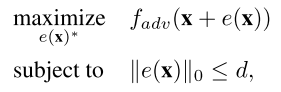• In the case of one-piexl attack $d=1$.
• Previous works commonly modify a part of all dimensions while in our approach only d dimensions are modified with the other dimensions of $e(\mathbf{x})$ left to zeros.
• Do the experiment on three different networks for classification (All Convolution Network, Network in Network, VGG16 Network)
• Some results for CIFAR-10 classification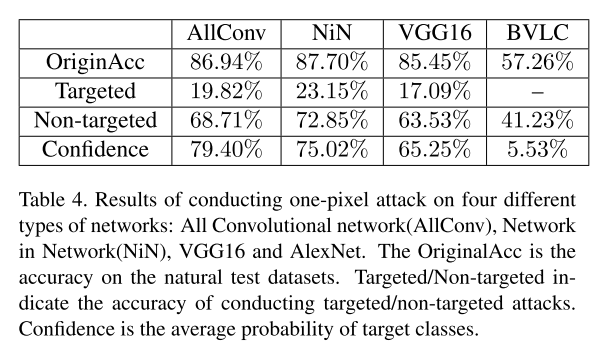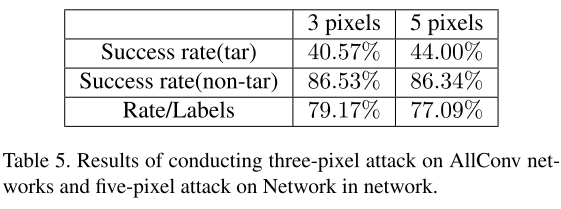### 2. Threat of Adversarial Attacks on Deep Learning in Computer Vision: A Survey

#### I Intro

• Szegedy et al.  first discovered an intriguing weakness of deep neural networks in the context of image classification.

• Moosavi-Dezfooli et al.  showed the existence of ‘uni- versal perturbations’ that can fool a network classifier on any image (see Fig. 1 for example)
• Similarly, Athalye et al.  demonstrated that it is possible to even 3-D print real- world objects that can fool deep neural network classifiers (see Section IV-C)
• Review parts (II~)

#### II Definition of terms

• Adversarial example/image is is a modified version of a clean image that is intentionally perturbed (e.g. by adding noise) to confuse/fool a machine learning tech- nique, such as deep neural networks.
• Adversarial perturbation is the noise that is added to the clean image to make it an adversarial example.
• Adversarial training uses adversarial images besides the clean images to train machine learning models.
• Adversary more commonly refers to the agent who creates an adversarial example. However, in some cases the example itself is also called adversary.
• Black-box attacks feed a targeted model with the adversarial examples (during testing) that are generated without the knowledge of that model. In some instances, it is assumed that the adversary has a limited knowledge of the model (e.g. its training procedure and/or its archi- tecture) but definitely does not know about the model. parameters. In other instances, using any information about the target model is referred to as ‘semi-black-box’ attack. We use the former convention in this article.
• Detector is a mechanism to (only) detect if an image is an adversarial example.
• Fooling ratio/rate indicates the percentage of images on which a trained model changes its prediction label after the images are perturbed.
• One-shot/one-step methods generate an adversarial per- turbation by performing a single step computation, e.g. computing gradient of model loss once. The opposite are iterative methods that perform the same computation multiple times to get a single perturbation. The latter are often computationally expensive.
• Quasi-imperceptible perturbations impair images very slightly for human perception.
• Rectifier modifies an adversarial example to restore the prediction of the targeted model to its prediction on the clean version of the same example.
• Targeted attacks fool a model into falsely predicting a specific label for the adversarial image. They are oppo- site to the non-targeted attacks in which the predicted label of the adversarial image is irrelevant, as long as it is not the correct label.
• Threat model refers to the types of potential attacks considered by an approach, e.g. black-box attack.
• Transferability refers to the ability of an adversarial example to remain effective even for the models other than the one used to generate it.
• Universal perturbation is able to fool a given model on ‘any’ image with high probability. Note that, universality refers to the property of a perturbation being ‘image- agnostic’ as opposed to having good transferability.
• White-box attacks assume the complete knowledge of the targeted model, including its parameter values, architecture, training method, and in some cases its training data as well.

#### III ADVERSARIAL ATTACKS (IN ‘laboratory settings’)

This part covers the literature in CV that introduces methods for adversarial attacks on deep learning and in laboratory settings. E.g. recognition, and their effectiveness is demostrated using standard datasets, e.g. MNIST.

• A. ATTACKS FOR CLASSIFICATION
• 1) BOX-CONSTRAINED L-BFGS
Szegedy et al. proposed to solve the following problem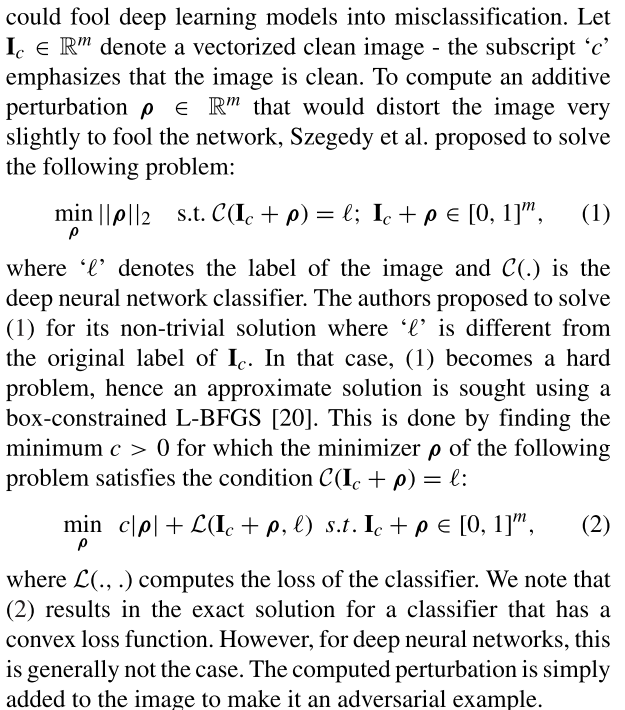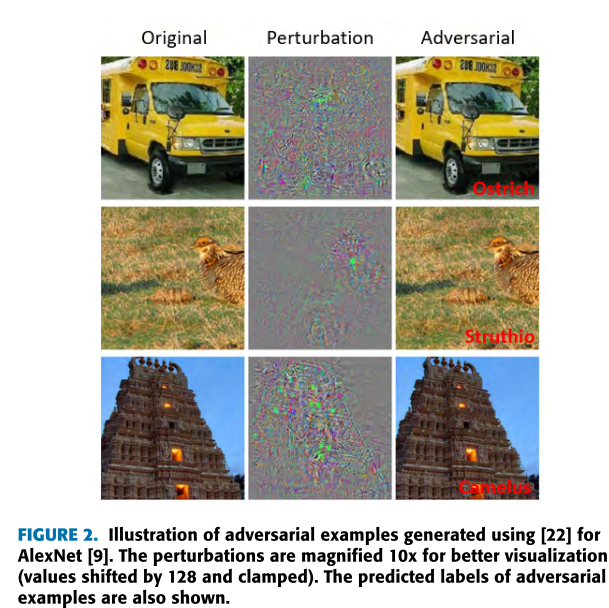• 2) FAST GRADIENT SIGN METHOD (FGSM)
To enable effec- tive adversarial training, Goodfellow et al.  developed a method to efficiently compute an adversarial perturbation for a given image by solving the following problem: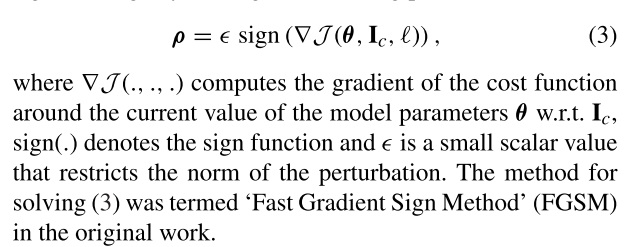Kurakin et al.  proposed a ‘one-step target class’ variation of the FGSM where instead of using the true label ? of the image in (3), they used the label ?target of the least likely class predicted by the network for $\mathbf{I}_c$. The computed perturbation is then subtracted from the original image to make it an adversarial example.
Miyato et al.  proposed a closely related method to compute the perturbation as follows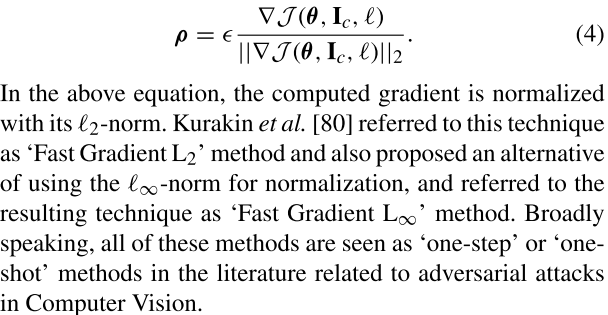• 3/) BASIC & LEAST-LIKELY-CLASS ITERATIVE METHODS
The one-step methods perturb images by taking a single large step in the direction that increases the loss of the classifier (i.e. one-step gradient ascent). An intuitive extension of this idea is to iteratively take multiple small steps while adjusting the direction after each step. , 
• 4/) JACOBIAN-BASED SALIENCY MAP ATTACK (JSMA)
Papernot et al.  also created an adversarial attack by restricting the l0-norm of the perturbations. Physically, it means that the goal is to modify only a few pixels in the image instead of perturbing the whole image to fool the classifie.
• 5/) ONE PIXEL ATTACK
An extreme case for the adversarial attack is when only one pixel in the image is changed to fool the classifier. Inter- estingly, Su et al.  claimed successful fooling of three different network models on 70.97% of the tested images by changing just one pixel per image. Su et al. computed the adversarial examples by using the concept of Differential
Evolution .
• 6/) CARLINI AND WAGNER ATTACKS (C&W)
A set of three adversarial attacks were introduced by Carlini and Wagner  in the wake of defensive distillation against the adversarial perturbations .
• 7/) DEEPFOOL
Moosavi-Dezfooli et al.  proposed to compute a minimal norm adversarial perturbation for a given image in an iterative manner.
9/) UPSET AND ANGRI
10/) HOUDINI
12/) MISCELLANEOUS ATTACKS

08-14448

#### 论文阅读 | Generating Fluent Adversarial Examples for Natural Languages

04-18181505-26110

#### 论文阅读：Generating Fluent Adversarial Examples for Natural Languages

09-201694

#### 【深度学习NLP论文笔记】《Adversarial Example For Natural Language Classification Problems》

09-047163

#### 【NLP】ACL-2019 录用论文

01-06694

11-08666

#### 对抗机器学习：Generating Adversarial Malware Examples for Black-box Attacks Based on GAN

12-14293

#### 论文阅读笔记三十：One pixel attack for fooling deep neural networks（CVPR2017）

08-293616

#### One pixel 对抗攻击_学习笔记

10-286481

#### 修改一个像素，就能让神经网络识别图像出错©️2020 CSDN 皮肤主题: 大白 设计师: CSDN官方博客点击重新获取扫码支付1.余额是钱包充值的虚拟货币，按照1:1的比例进行支付金额的抵扣。
2.余额无法直接购买下载，可以购买VIP、C币套餐、付费专栏及课程。余额充值